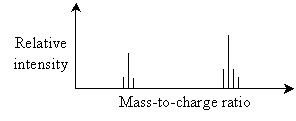NMR, Mass Spectrometry, and Infrared (IR) Spectroscopy

NMR, Mass Spectrometry, and Infrared (IR) Spectroscopy

Introduction

Although the naked eye can provide some help, many chemicals are indistinguishable by their appearance. Spectroscopy and spectrometry, however, allow chemists to determine the structures of compounds using sophisticated instruments.

Key Terms

• Spectroscopy
• Spectrometer
• Infrared (IR) spectroscopy
• Quantization
• Quantum/quanta
• Fingerprint region
• Nuclear magnetic resonance (NMR)
• Chemical shift
• Mass spectrometry

Objectives

• Introduce the underlying concepts behind three major types of chemical instruments
• Recognize the physical principles that allow chemists to use NMR spectroscopy, mass spectrometry, and IR spectroscopy to identify the structure and composition of an unknown sample

You may have wondered how chemists can differentiate one compound from another. Sure, some chemicals have different colors, odors, or textures compared with others, but this is seldom sufficient to identify the structure of those compounds. We have seen that different chemicals-depending on the functional groups they include-behave differently under certain conditions. And historically, this was how chemicals were identified: chemists tested compounds through reactions, attempting to identify unknown compounds by comparing the results with known reactions. They were then able to propose a structure that could possibly be confirmed through further testing by way of reactions.

With the advent of computers and other sophisticated machines, chemists have created and been able to use a wealth of instruments to improve their ability to identify chemical compounds. We will ever so briefly examine three methods: nuclear magnetic resonance (NMR) spectroscopy, mass spectrometry, and infrared spectroscopy. Each of these methods could encompass a course of its own, so we will not be able to go into great detail on any particular one of them. We will, however, discuss the general principles that underlie each one, giving you a foundation for future investigation of these techniques and the instruments that use them.

Spectroscopy Overview

Spectroscopy (and, similarly, spectrometry) is the measurement and analysis of the effect of a compound on light that is incident on it. A spectrometer is an instrument for performing spectroscopy. The underlying concept, however, is (fundamentally, anyway) simpler than the jargon would indicate.

Spectroscopy involves passing light (electromagnetic radiation) through a sample and looking at what frequencies of light are transmitted. The diagram below offers a simple view of how spectroscopy works.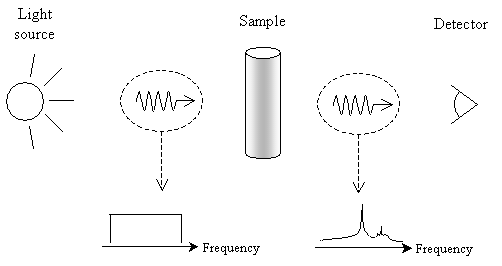When light is passed through the sample, certain frequencies (or colors, when in the visible portion of the spectrum) are absorbed. By looking at what portions of the spectrum are absorbed or transmitted and comparing these results with those of known chemicals, chemists can determine the composition and structure of unknown samples. Of course, the instruments needed to perform these analyses are complicated (and beyond the scope of this article), but the fundamental principle is simple, as illustrated above. We now turn to several types of spectroscopy, highlighting some key principles of how chemists use them in analyzing chemical compounds.

Infrared Spectroscopy

Infrared (IR) spectroscopy, as the name indicates, uses infrared radiation (a band of frequencies below the visible portion of the spectrum) to analyze a sample. Instead of describing the light in terms of frequencies, however, IR spectroscopy deals with wavenumbers (the reciprocal of wavelength). Frequency (ν) and wavenumber (k) are related to the speed of light (c, or about 3 108 meters per second) by the following formula: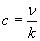where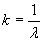Note here that λ is the wavelength. Typically, IR spectra are in the wave number range of 4,000 to 500 cm-1 (this range is useful for identifying functional groups and overall compounds) and may be displayed as a graph of percent transmittance versus wave number. An example plot is shown below (the spectrum doesn't necessarily conform to a real chemical, however).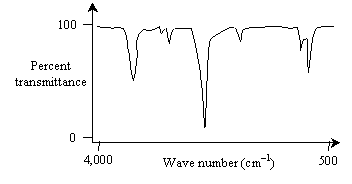The "valleys" in the spectrum, because they are much less than 100% transmittance, represent wave numbers that the sample absorbs (more or less strongly). But what does it mean when a compound absorbs a particular wave number of electromagnetic (IR, in this case) radiation?

The answer to this question lies in the "flexibility" of bonds between atoms. Two atoms in a compound reside at a given distance from each other that represents the point at which the various forces (among the positively charged nuclei and negatively charged electrons, mainly) are balanced. But these atoms can be disturbed in such a manner that this bond length changes, causing an oscillation (like what you would see in a spring). The result is vibrational modes in the molecule, and these modes are quantized. Please note how electrons can only occupy certain discrete energy states: this is an example of quantization. Likewise, electromagnetic energy, rather than being emitted in continuous "streams," is emitted in energy "packets" (or quanta-plural for quantum) with a discrete range of possible energy values. Furthermore, the energy of a quantum of electromagnetic energy (or "light") is directly proportional to its frequency by the constant h-Planck's constant (6.63 10-34 J·s). Thus,

E =

In the same manner, vibrational modes of a molecule only take on certain discrete energy values.

Consider the hydrogen atoms in dichloromethane (shown below). If, for the sake of argument, we hold the carbon and two chlorine atoms in fixed positions, then we can imagine the hydrogen atoms vibrating in a variety of ways.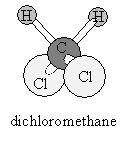The hydrogen atoms could then vibrate by "bending" or "stretching" the bonds: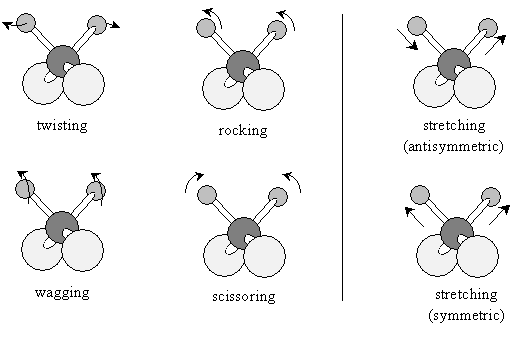Different compounds will absorb different wave numbers of infrared radiation to cause certain of these vibrational modes. The 4,000 to 1,500 cm-1 range is largely indicative of the presence of certain functional groups in a molecule; the 1,500 to 500 cm-1 range (called the fingerprint region) can help identify a particular compound because the spectra are the most unique to each compound in this range. IR spectroscopy is a powerful tool for identifying different functional groups in a molecule.

Nuclear Magnetic Resonance Spectroscopy

Nuclear magnetic resonance (NMR) spectroscopy takes advantage of the spin states of protons (and, to some extent, other nuclei) to identify a compound. Electrons can have one of two spin quantum states, which we designated as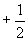and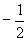. These spin states have equal energy in the absence of an applied magnetic field. Likewise, protons also have two spin states (with the same designations) of equal energy. When a magnetic field is applied, the protons tend to align their magnetic moments (a vector associated with the "spin" of the particle) parallel with the field-this is the low-energy state. But the protons can also align their magnetic moments antiparallel to the magnetic field-this is the high-energy state. The stronger the magnetic field, the larger the energy difference between the parallel and antiparallel aligned states.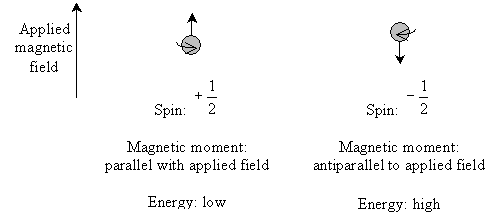To complicate matters (and to make NMR spectroscopy possible), electrons in the molecule cause "shielding" effects that reduce the magnitude of the magnetic field felt by the protons. Thus, for instance, a compound containing a highly electronegative atom would tend to leave the hydrogen nuclei (protons) more exposed to the magnetic field because the electronegative atom "hogs" the electrons. Conversely, a compound without a highly electronegative atom would tend to allow greater shielding of protons by electrons. Less shielded protons (which are more exposed to the applied field) thus have a greater difference between high-energy and low-energy spin states, whereas more-shielded protons have a lesser difference between the spin states.

As with IR spectroscopy, NMR spectroscopy passes light through a sample and looks at the spectrum that is transmitted. In this case, however, absorption occurs at frequencies corresponding to the energy difference between two spin states of a proton in the compound. A slightly revised (but still simple) view of NMR spectroscopy is depicted below.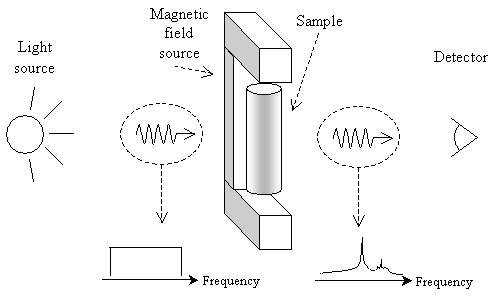NMR spectra are typically displayed as a series of peaks, such as the sketch shown below (not necessarily representative of any existing compound).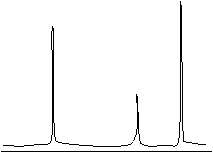Instead of displaying the peaks on a frequency (or wave number) scale, however, the horizontal axis is often displayed according to chemical shift, which is a number proportional to the difference in frequency between the sample peak and a reference peak (typically tetramethylsilane, or (CH3)4Si). Although we will not delve much further into the details of NMR spectra, we can note that we can interpret these spectra by way of the number of peaks (indicating the number of different proton types in the compound), the peak heights (indicating the relative numbers of proton types), and peak splitting (indicating the number of protons near the one creating the peak). Thus, NMR is useful for determining the structure of a sample.

Mass Spectrometry

Although it is not truly a type of spectroscopy, mass spectrometry is nevertheless another instrumental method that chemists use to analyze compounds. Fundamentally, mass spectrometry involves ionization of a sample through bombarding it with high-energy electrons. When these electrons collide with a molecule in the sample, they can dislodge an electron, creating either a cation radical or a cation and a neutral radical.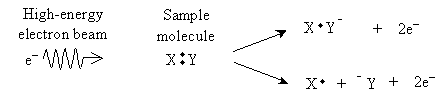The result is a number of charged particles that can be accelerated by an electric field and then deflected by a magnetic field as shown in the diagram sketched below.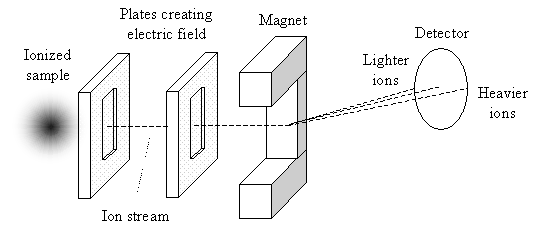Lighter ions (relative to their respective charges) will be deflects more by the magnetic field than will heavier ions (also relative to their respective charges). Thus, the detector can identify different ion masses (actually, mass-to-charge ratios) by the amount of deflection they undergo, and chemists can use this information to identify the type of compound in the sample. An example mass spectrometry spectrum is shown below. (Again, this spectrum is for illustration; it does not necessarily correspond to an actual compound.) Mass-to-charge ratios are often calculated as the atomic weight (essentially the number of protons and neutrons in the nucleus of an atom) of the entire molecule divided by the charge (in the number of missing electrons).